# How to Convert a Categorical Variable to Numeric in Pandas and Scikit-LearnWhenever we feed text into a computer, it decodes it into 0s and 1s which cannot be directly understood by humans. It interprets these numbers as instructions for displaying text, sound, image, etc., which are meaningful to people. Similarly, when we send data to any machine learning (ML) model, we need to do it in the proper format since algorithms only understand numbers. These categorical variables also contain valuable pieces of information about the data. In this article, we will learn how to encode categorical variables to numeric with Pandas and Scikit-learn.

## Ways to encode categorical variables

Categorical variables are generally addressed as ‘strings’ or ‘categories’ and are finite in number. Here are a few examples:

1. The city where a person lives: San Francisco, Chicago, Las Vegas, Seattle, etc.

2. The department a person works in: Marketing, Sales, Human Resource, etc.

3. The highest degree a person has: High school, Bachelor’s, Master’s, PhD, etc.

There are two types of categorical data, ordinal and nominal.

• Ordinal data: The values follow some natural order. When encoding ordinal data, we need to retain the information regarding the order in which the category is provided. The sequence is very important. For example, the degree of a person gives us important information about his qualifications. We cannot randomly assign numbers to these degrees.

• Nominal data: The categories cannot be ordered in any meaningful way. In nominal data, there is an assumption of order present. We just have to consider the presence and absence of a feature. For instance, in the above example, knowing a person’s city is important. It is equally so if the person likes Chicago or Las Vegas.

Now that we have knowledge about categorical variables, let’s look at the options for encoding them using Pandas and Scikit-learn.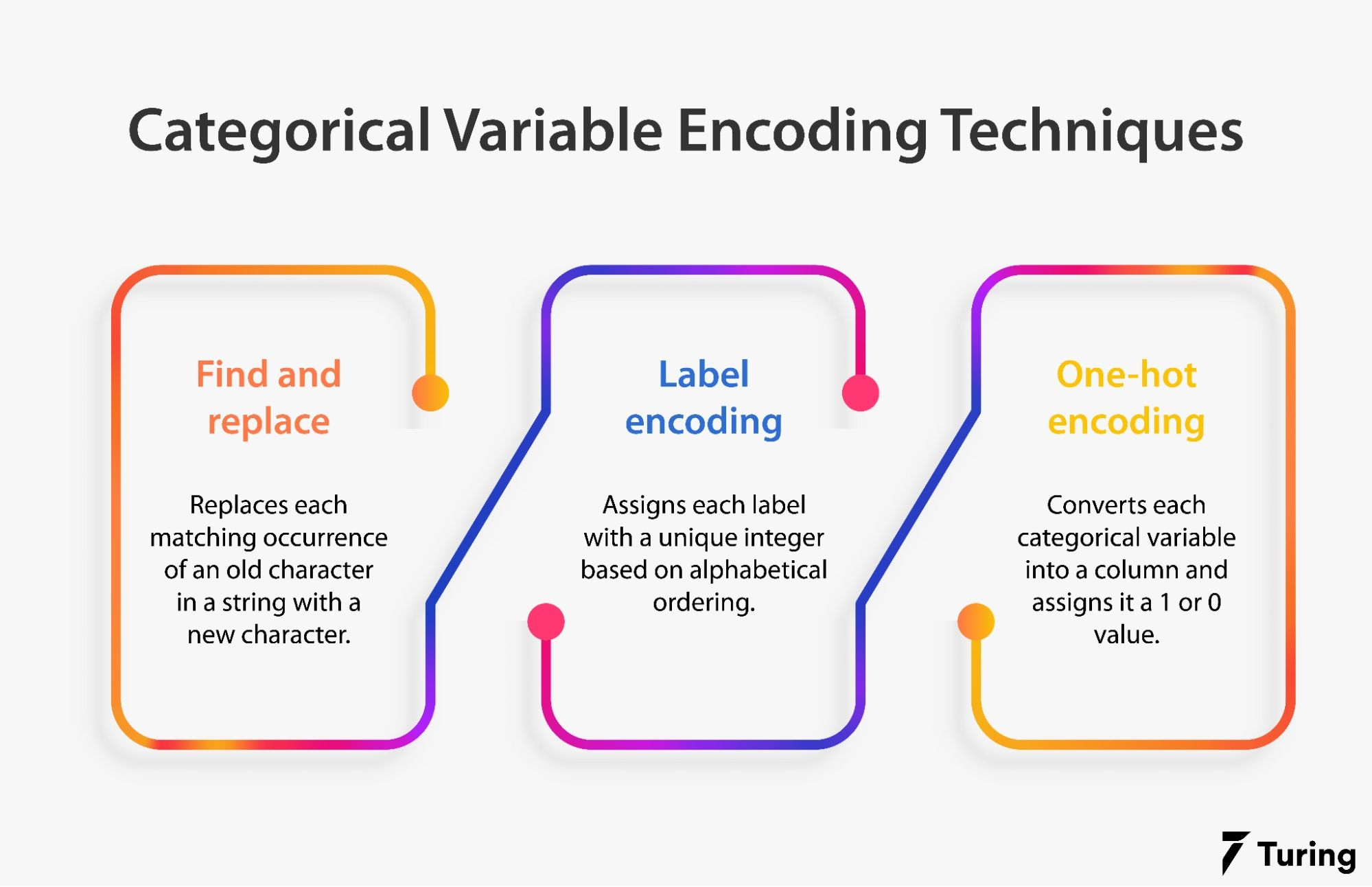### Find and replace

The simplest method of encoding categorical data is with find and replace. The replace() method replaces each matching occurrence of the old character in the string with the new character.

Here’s how it works:

Suppose there is a column named “number of cylinders” in a dataset and the highest cylinder a car can have is 4. The values this column contains cannot exceed 4. However, the problem is that all these values are written in text, such as “two”, “one”, etc. What we can do is directly replace these text values with their numeric equivalent by using the ‘replace’ function provided by Pandas.

``````numeric_var = {“num_cylinders”: {“four”:4, “three”:3, “two”:2, “one”:1}}
df = df.replace(numeric_var)

``````

Here, we are creating a mapping dictionary that will map all the text values to their numeric values. This approach is very useful when dealing with ordinal data because we need to maintain the sequence.

In the above example of “a person’s degree”, we can map the highest degree to a greater number and the lowest degree to the lowest number.

### Label encoding

In this approach, each label is assigned a unique integer based on alphabetical ordering. We can implement this using the Scikit-learn library.

``````import pandas as pd
import numpy as np

``````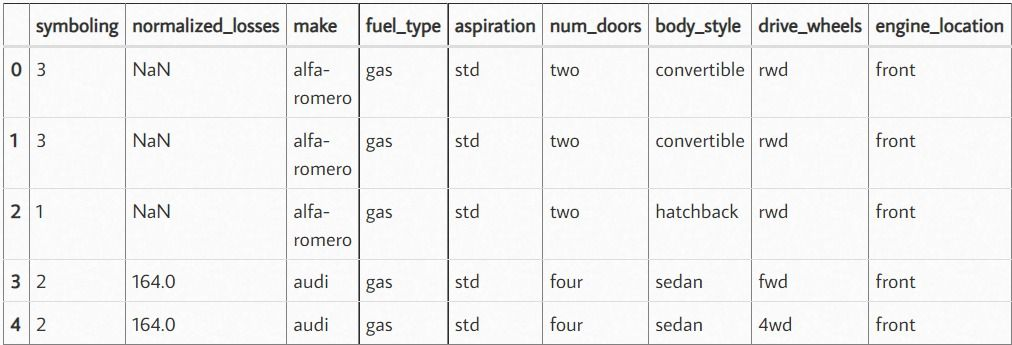This dataset contains some null values, so it’s important to remove them.

Let’s look at the data type of these features:

``````df.info()

``````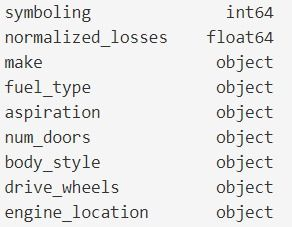We can see that almost all the variables are represented by the object data type, except the “symboling” column.

Let’s encode the “body_style” column:

``````#import label encoder
from sklearn import preprocessing
#make an instance of Label Encoder
label_encoder = preprocessing.LabelEncoder()
df[‘’body_style”] = label_encoder.fir_transform(data[‘body_style’)

``````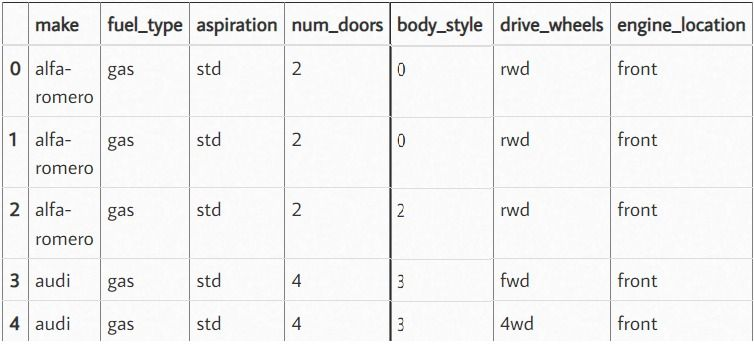Image source: Practical Business Python

Since label encoding uses alphabetical ordering, “convertible” has been encoded with 0, “hatchback” has been encoded with 2, and “sedan” with 3. There must be another category in body_style that was encoded with 1.

If we look at the “body_style” column, we will notice that it does not have any order. If we perform label encoding on it, we will see that the column is ranked based on the alphabets. Due to this order, the model may capture some hypothetical relationship.

### One-hot encoding

We generally use one-hot encoding to solve the disadvantage of label encoding. The strategy is to convert each category into a column and assign it a 1 or 0 value. It is a process of creating dummy variables.

Let’s see how we can implement it in Python:

``````Import pandas as pd

#Creating a dataframe

Df = pd.Dataframe({‘City’ :

``````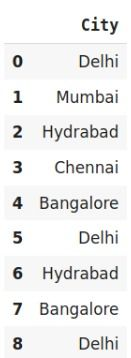``````From sklearn.preprocessing, import OneHotEncoder.
#creating instance of one hot encoder
Onehotencoder = OneHotEncoder()
# fir_transform expects 2-D array hence we need to reshape the data from 1-D to 2-D.
df =df.values.reshape(-1,1).toarray()
X = onehotencoder.fit_transform(df)
df_onehot = pd.Dataframe(X,columns=[‘City_’+str(int(i)) for i in range (df.shape)])

``````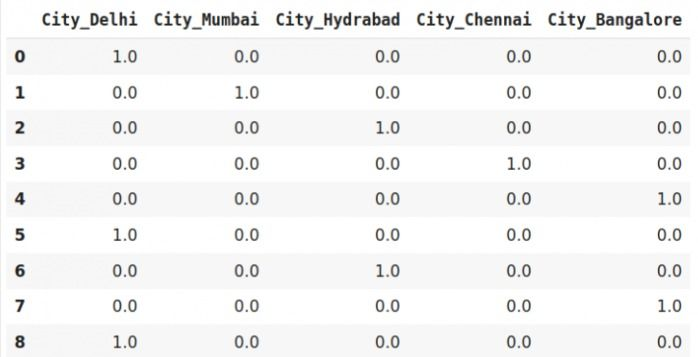We can see from the table above that all the unique categories were assigned a new column. If a category is present, we have 1 in the column and 0 for others.

Since the data is sparse, it results in a dummy variable trap as the outcome of one variable can be predicted with the help of the remaining variables. This problem occurs when the variables are highly correlated to each other. It also leads to a collinearity problem which causes issues in various regression models.

There’s another problem with this method: if there are many unique categories and we want to encode them, we will have many extra columns. This will eventually increase the model complexity and time as it will take longer to analyze the relationship between the variables.

## Converting categorical data to numerical data using Pandas

The following are the methods used to convert categorical data to numeric data using Pandas.

### Method 1: Using get_dummies()

Syntax:

``````pandas.get_dummies(data, prefix=None, prefix_sep=’_’, dummy_na=False,
columns=None, sparse=False, drop_first=False, dtype=None)

#import libraries
import pandas as pd
# reading the csv file

``````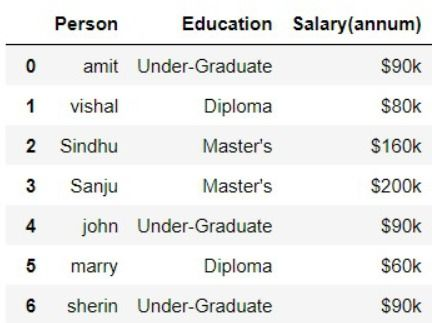Image source: GeeksforGeeks

``````# using get_dummies on education column
encoded = pd.get_dummies(df.Education)
# Concatenate the dummies to original dataframe
merged_data = pd.concat([df, encoded], axis='columns')
# dropping the original column which was not encoded
# print the dataframe
print(merged_data)

``````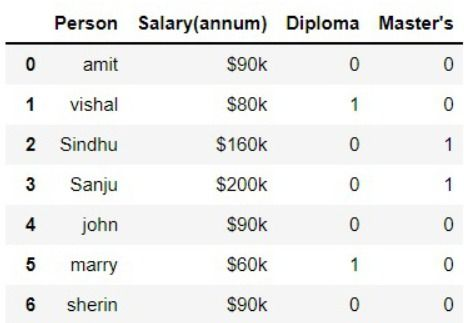Image source: GeeksforGeeks

### Method 2: Using replace()

Syntax:

``````replace(to_replace=None, value=None, inplace=False, limit=None, regex=False,

``````

Convert the same data using a different approach: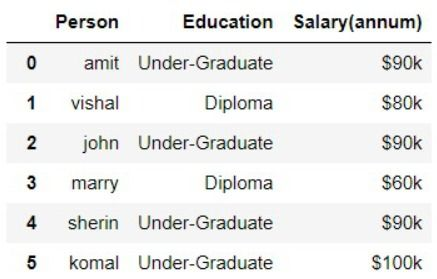Image source: GeeksforGeeks

``````#importing libraries
import pandas as pd
# reading the csv file
# replacing values
[0, 1], inplace=True)

``````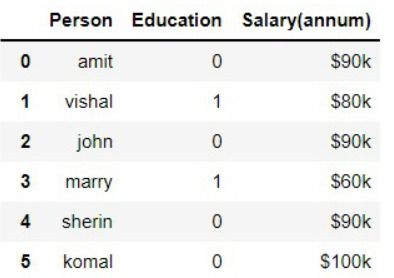Image source: GeeksforGeeks

## Converting categorical data to numerical data using Scikit-learn

Converting categorical data to numerical data in Scikit-learn can be done in the following ways:

### Method 1: Label encoding

Let’s implement this on different data and see how it works.

``````#importing the libraries
import pandas as pd
from sklearn.preprocessing import LabelEncoder
#reading the csv file

``````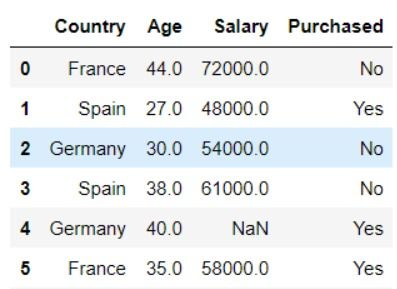Image source: GeeksforGeeks

``````#making instance of labelencoder()
le = LabelEncoder()
encoded = le.fit_transform(df[‘Purchased’])
print(‘encoded’)

``````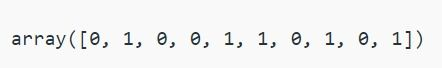``````# removing the original column 'Purchased' from df
df.drop("Purchased", axis=1, inplace=True)

# Appending the array to our dataFrame
df["Purchased"] = encoded
# printing Dataframe

``````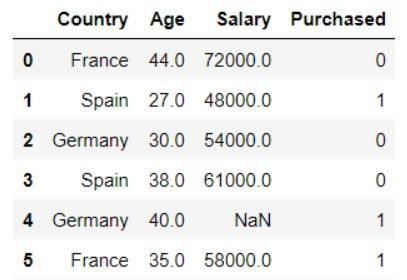Image source: GeeksforGeeks

### Method 2: One-hot encoding

``````#importing the libraries
import pandas as pd
from sklearn.preprocessing import OneHotEncoder
#reading the csv file
#making instance of labelencoder()
enc = OneHotEncoder()
enc.fit(df[‘Purchased’])
encoded = enc.transform(df[‘Purchased’])
# removing the original column 'Purchased' from df
df.drop("Purchased", axis=1, inplace=True)
# Appending the array to our dataFrame
df["Purchased"] = encoded

``````

## Which encoding technique to use?

In order to know when to use which encoding technique, we need to understand our data well. We then need to decide which model to apply.

For example, if there are more than 15 categorical features and we decide to use the support vector machine (SVM) algorithm, the training time might increase as SVM is slow. Feeding it many features separately adds to the model’s complexity and training time.

Below are some key points to note when choosing an encoding technique:

### Use find and replace method

1. When preserving the sequence is important as this approach helps maintain the order.

2. When the data is ordinal or represents some kind of intensity. For example, if the values of a variable are small, medium, and big, we can map them to 1, 2, and 3 respectively.

### Use one-hot encoding

1. When the categorical feature has no order or is not ordinal. For example, the city where a person is in is not ordinal so one-hot encoding can be used for such types of columns.

2. When the number of unique categorical features is less. This is because more features increase the model’s complexity and training time.

### Use label encoding

1. When the categorical feature has some order or is ordinal. For example, an army’s position is ordinal and the highest position has a high number.

2. When the number of categories is large.

We have explored the various ways to encode categorical data along with their issues and suitable use cases. To summarize, encoding is a crucial and unavoidable part of feature engineering. It’s important to know the advantages and limitations of all the methods used too so that the model can learn properly.

## Author

•### Turing

Author is a seasoned writer with a reputation for crafting highly engaging, well-researched, and useful content that is widely read by many of today's skilled programmers and developers.

## Frequently Asked Questions

This can be done using get_dummies() and replace methods.

This can be done using label encoding and one-hot encoding.

In terms of modeling, no, as we cannot feed categorical inputs to a model directly. However, when it comes to encoding these categorical variables via Scikit-learn, there are various ways to do it, such as with label encoder and one-hot encoder.### Press

What's up with Turing? Get the latest news about us here.### Blog

Know more about remote work.
Checkout our blog here.### Contact

Have any questions?
We'd love to hear from you.

#### Hire and manage remote developers

Tell us the skills you need and we'll find the best developer for you in days, not weeks.